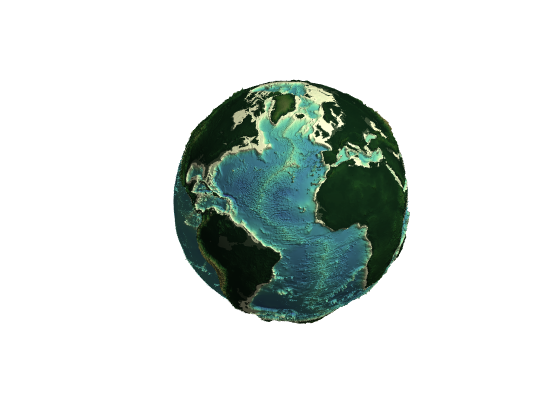# globesurf documentation

The globesurf function plots georeferenced data on a globe where values in matrix Z are plotted as heights above the globe.

## Syntax

```globesurf(lat,lon,Z)
globesurf(lat,lon,Z,C)
globesurf(...,'exaggeration',exaggerationFactor)
h = globesurf(...)```

## Description

globesurf(lat,lon,Z) plots the georeferenced values given by Z at heights above a globe of radius 6371, where 6371 corresponds to the average radius of the Earth in kilometers. The inputs lat and lon are the same size as Z and can be defined for arbitray domains using the meshgrid function.

globesurf(lat,lon,Z,C) specifies the colors of the georeferenced values either by a matrix the same size as Z or as an m-by-n-by-3 array of RGB triplets, where Z is m-by-n.

globesurf(...,'exaggeration',exaggerationFactor) scales the plotted height of the georeferenced values by a factor specified by exaggerationFactor.

globesurf(...,'radius',GlobeRadius) plots the georeferenced values as heights above a globe of radius specified by GlobeRadius.

h = globesurf(...) returns the handle h of the plotted objects.

## Example 1

For this example, plot color-scaled global topography. use cdtgrid to create a quarter-degree grid, and topo_interp to get the corresponding topography. Here's the data we'll be plotting:

```% Create the 1/4 degree grid:
[Lat,Lon] = cdtgrid(1/4);

% Get the corresponding topography:
Z = topo_interp(Lat,Lon);
```

Plot the surface topography, exaggerated by a factor of 50. Set the colormap with cmocean using the 'pivot' option to put zero in the middle of the colormap.

```figure
globesurf(Lat,Lon,Z,'exag',50)
axis tight
cmocean('topo','pivot')
```Set the viewing angle and adjust the lighting position and material reflectance:

```view(60,20)
camlight
material dull
```## Example 2

Plot deviation of the Earth radius from the average Earth radius exaggerated million-fold. Use earthradius to get the ellipsoidal radius of the Earth:

```[lat,lon] = cdtgrid;

dR = R - 6371;

figure
globesurf(lat,lon,dR,'exag',1e6)
axis tight

% Adjust the view:
view(10,20)
camlight
material dull
```## Author Info

This function and supporting documentation were written by Natalie S. Wolfenbarger for the Climate Data Toolbox for Matlab, 2019.